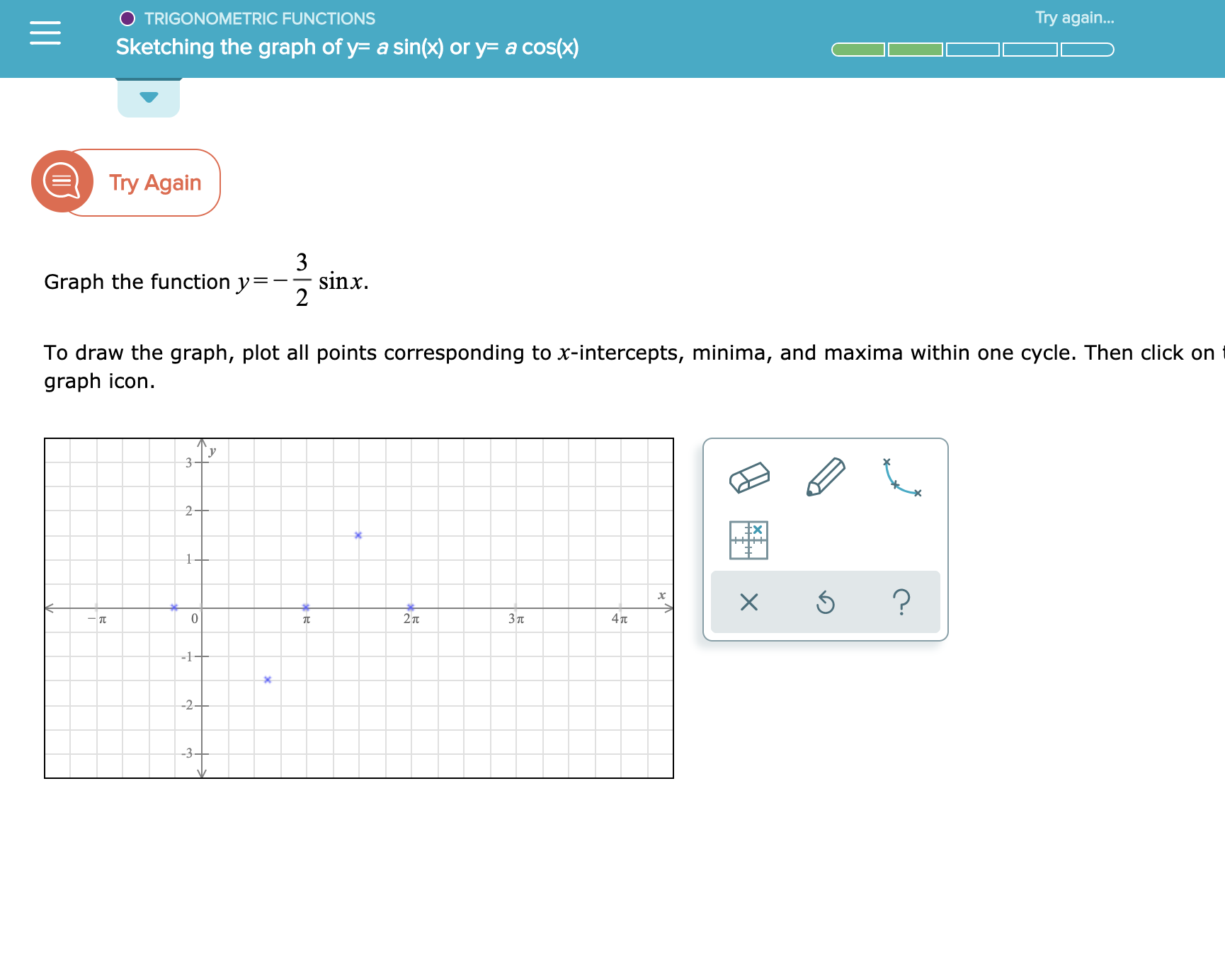# Try again... TRIGONOMETRIC FUNCTIONS Sketching the graph of y a sin(x) or y= a cos(x) Try Again 3 sinx Graph the function y 2 To draw the graph, plot all points corresponding to x-intercepts, minima, and maxima within one cycle. Then click on graph icon 2 1 ? 2 T Зп 47t -1+ -2 3-

Question

See attachmenthelp_outlineImage TranscriptioncloseTry again... TRIGONOMETRIC FUNCTIONS Sketching the graph of y a sin(x) or y= a cos(x) Try Again 3 sinx Graph the function y 2 To draw the graph, plot all points corresponding to x-intercepts, minima, and maxima within one cycle. Then click on graph icon 2 1 ? 2 T Зп 47t -1+ -2 3- fullscreen